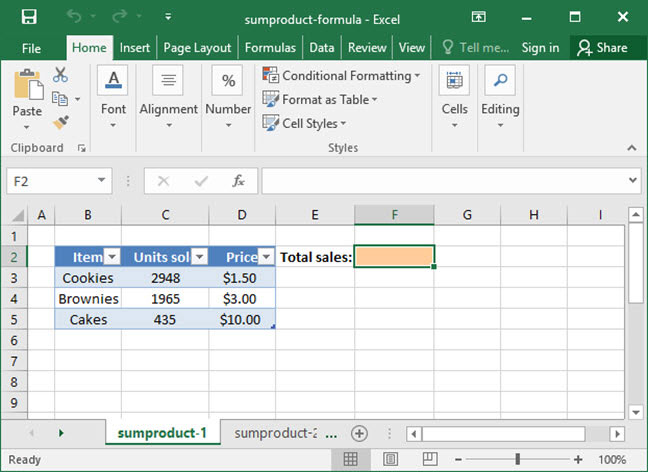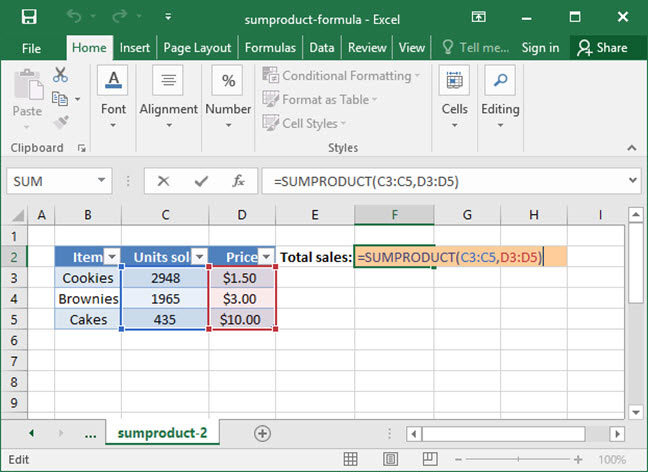# Using SUMPRODUCT in Formulas

SUMPRODUCT is one of Excel's most interesting and advanced mathematical formulas. At a high level, it is used to multiply two arrays of numbers, and then add all of the resulting values together. That may not seem like a particularly useful thing to do, but it turns out that SUMPRODUCT can be used for many things beyond simple multiplication and addition. Mastry of this formula — and its use in more advanced functions — can go a long way towards making your spreadsheets automated and flexible.

Read on to find out how to use SUMPRODUCT in a formula yourself.

## The SUMPRODUCT formula

The syntax for SUMPRODUCT is as follows:

`=SUMPRODUCT(array_or_range_1, array_or_range_2, array__or_range_3 (optional)...)`

SUMPRODUCT takes as its arguments a set of arrays. An array is basically just a list of numerical values. For example, the array {1, 2, 3} contains three values: 1, 2, and 3. When we use arrays in Excel, we enclose them in a set of curly brackets ({ }) to let the program know that we are talking about an array rather than a list of arguments to a function.

Instead of arrays, SUMPRODUCT can also take ranges as its arguments — like the cells C3:C6.

When SUMPRODUCT is called, it multiplies the contents of all its arrays together, and then adds the results. The first number of the first array provided is multiplied with the first number of the second array provided, then with the first number of the third array provided, etc. This value is then stored as Excel moves on to multiplying the second number of the first array, with the second number of the second array, with the second number of the third array, etc. Once each number of each array has been multiplied together, Excel adds the results.

To make things a little bit more clear, let's look at a practical example and examine how Excel executes it:

`=SUMPRODUCT({1, 2, 3}, {4, 5, 6})Step 1: =((1*4) + (2*5) + (3*6))Step 2: =(4 + 10 + 18)Output: 32`

As you can see, Excel multiplies together the matching values in each array — 1 and 4; 2 and 5; and 3 and 6 — and then adds the resulting values together.

Note that we can use the SUMPRODUCT function with as many arrays as we want, but they all have to be the same length so that Excel knows exactly which numbers to multiply together. Here's another example, in which we use SUMPRODUCT with three arrays:

`=SUMPRODUCT({1, 2, 3}, {4, 5, 6}, {0, 2, 1})Step 1: =((1*4*0) + (2*5*2) + (3*6*1))Step 2: =(0 + 20 + 18)Output: 38`

## SUMPRODUCT used practically with a cell range

Per the above, SUMPRODUCT can also be used with a range of cells. Let's take a look at an example of it used in a real-life formula.

Take a look at the below spreadsheet, which lists SnackWorld's daily product sales by category, item price, and number of units sold.A SnackWorld analyst wants to figure out total sales for the day. She could do this by multiplying together the Number sold and Unit price columns for each category, then summing the results. But there's an easier way to solve the problem using SUMPRODUCT:`=SUMPRODUCT(C3:C5, D3:D5)Step 1: =((2,948*\$1.50) + (1,965*\$3.00) + (435*\$10.00))Step 2: =(\$4,422.00 + \$5,895.00 + \$4,350.00)Output: \$14,667.00`

As expected, SUMPRODUCT first multiplies together the matching values in each range provided — 2948 and \$1.50; 1965 and \$3.00; and 435 and \$10.00 — and then adds the resulting values together for a grand total of \$14,667.00. We have successfully calculated the grand total SnackWorld dailiy sales with one formula and no additional columns!

## SUMPRODUCT used to calculate weighted averages

Another frequent use of the SUMPRODUCT formula is to calculate weighted averages. Check out our tutorial on weighted averages for more information.

Now that you've got a basic handle on SUMPRODUCT, put it into action in your formulas!

## Save an hour of work a day with these 5 advanced Excel tricks

Work smarter, not harder. Sign up for our 5-day mini-course to receive must-learn lessons on getting Excel to do your work for you.

• How to create beautiful table formatting instantly...
• Why to rethink the way you do VLOOKUPs...
• Plus, we'll reveal why you shouldn't use PivotTables and what to use instead...